# Calculating week number in Excel (WEEKNUM function)

While Microsoft Excel provides an array of functions to work with weekdays, months and years, only one is available for weeks - the WEEKNUM function. So, if you are looking for a way to get a week number from a date, WEEKNUM is the function you want.

In this short tutorial, we will briefly talk about the syntax and arguments of Excel WEEKNUM, and then discuss a few formula examples demonstrating how you can use the WEEKNUM function to calculate week numbers in your Excel worksheets.

## Excel WEEKNUM function - syntax

The WEEKNUM function is used in Excel to return the week number of a specific date in the year (a number between 1 and 54). It has two arguments, the 1st is required and the 2nd is optional:

WEEKNUM(serial_number, [return_type])
• Serial_number - any date within the week whose number you are trying to find. This can be a reference to a cell containing the date, a date entered by using the DATE function or returned by some other formula.
• Return_type (optional) - a number that determines on which day the week begins. If omitted, the default type 1 is used (the week beginning on Sunday).

Here is a complete list of the `return_type` values supported in WEEKNUM formulas.

 Return_type Week begins on 1 or 17 or omitted Sunday 2 or 11 Monday 12 Tuesday 13 Wednesday 14 Thursday 15 Friday 16 Saturday 21 Monday (used in System 2, please see the details below.)

In the WEEKNUM function, two different week numbering systems are used:

• System 1. The week containing January 1 is considered the 1st week of the year and is numbered week 1. In this system, the week traditionally starts on Sunday.
• System 2. This is the ISO week date system that is part of the ISO 8601 date and time standard. In this system, the week starts on Monday and the week containing the first Thursday of the year is considered week 1. It is commonly known as the European week numbering system and it is used mainly in government and business for fiscal years and timekeeping.

All of the return types listed above apply to System 1, except for return type 21 that is used in System 2.

Note. In Excel 2007 and earlier versions, only options 1 and 2 are available. Return types 11 through 21 are supported in Excel 2010 and Excel 2013 only.

## Excel WEEKNUM formulas to convert date to week number (from 1 to 54)

The following screenshot demonstrates how you can get week numbers from dates with the simplest `=WEEKNUM(A2)` formula: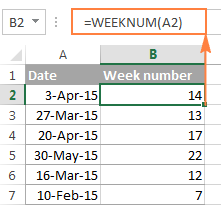In the above formula, the `return_type` argument is omitted, which means that the default type 1 is used - the week beginning on Sunday.

If you'd rather begin with some other day of the week, say Monday, then use 2 in the second argument:

`=WEEKNUM(A2, 2)`

Instead of referring to a cell, you can specify the date directly in the formula by using the DATE(year, month, day) function, for example:

`=WEEKNUM(DATE(2015,4,15), 2)`

The above formula returns 16, which is the number of the week containing April 15, 2015, with a week beginning on Monday.

In real-life scenarios, the Excel WEEKNUM function is rarely used on its own. Most often you would use it in combination with other functions to perform various calculations based on the week number, as demonstrated in further examples.

## How to convert week number to date in Excel

As you have just seen, it's no big deal to turn a date into a week number using the Excel WEEKNUM function. But what if you are looking for the opposite, i.e. converting a week number to a date? Alas, there is no Excel function that could do this straight away. So, we will have to construct our own formulas.

Supposing you have a year in cell A2 and a week number in B2, and now you want to calculate the Start and End dates in this week.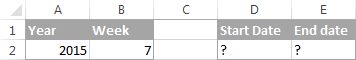Note. This formula example is based on ISO week numbers, with a week starting on Monday.

The formula to return the Start date of the week is as follows:

`=DATE(A2, 1, -2) - WEEKDAY(DATE(A2, 1, 3)) + B2 * 7`

Where A2 is the year and B2 is the week number.

Please note that the formula returns the date as a serial number, and to have it displayed as a date, you need to format the cell accordingly. You can find the detailed instructions in Changing date format in Excel. And here is the result returned by the formula: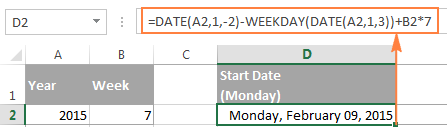Of course, the formula to convert a week number to a date is not trivial, and it may take a while to get your head round the logic. Anyway, I will do my best to provide meaningful explanation for those who are curious to get down to the bottom.

As you see, our formula consists of 2 parts:

• `DATE(A2, 1, -2) - WEEKDAY(DATE(A2, 1, 3))` - calculates the date of the last Monday in the previous year.
• `B2 * 7` - adds the number of weeks multiplied by 7 (the number of days in a week) to get the Monday (start date) of the week in question.

In the ISO week numbering system, week 1 is the week containing the first Thursday of the year. Consequently, the first Monday is always between December 29 and January 4. So, to find that date, we have to find the Monday immediately before January 5.

In Microsoft Excel, you can extract a day of week from a date by using the WEEKDAY function. And you can use the following generic formula to get Monday immediately before any given date:

=date - WEEKDAY(date - 2)

If our ultimate goal were to find Monday immediately before the 5th of January of the year in A2, we could use the following DATE functions:

`=DATE(A2,1,5) - WEEKDAY(DATE(A2,1,3))`

But what we actually need is not the first Monday of this year, but rather the last Monday of the previous year. So, you have to subtract 7 days from January 5 and hence you get -2 in the first DATE function:

`=DATE(A2,1,-2) - WEEKDAY(DATE(A2,1,3))`

Compared to the tricky formula you have just learned, calculating the End date of the week is a piece of cake :) To get Sunday of the week in question, you simply add 6 days to the Start date, i.e. `=D2+6`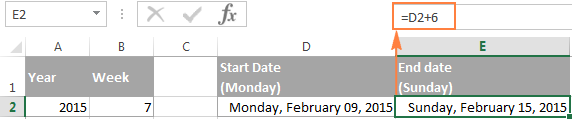Alternatively, you could add 6 directly in the formula:

`=DATE(A2, 1, -2) - WEEKDAY(DATE(A2, 1, 3)) + B2 * 7 + 6`

To make sure the formulas always deliver the right dates, please have a look at the following screenshot. The Start Date and End Date formulas discussed above are copied across column D and E, respectively: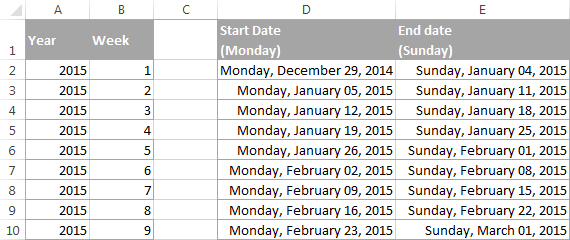### Other ways to convert week number to date in Excel

If the above formula based on the ISO week date system does not meet your requirements, try one of the following solutions.

#### Formula 1. A week containing Jan-1 is week 1, Mon-Sun week

As you remember, the previous formula works based on the ISO date system where the first Thursday of the year is considered week 1. If you work based on a date system where the week containing the 1st of January is considered week 1, use the following formulas:

Start date:

`=DATE(A2,1,1) - WEEKDAY(DATE(A2,1,1),2) + (B2-1)*7 + 1`

End date:

`=DATE(A2,1,1)- WEEKDAY(DATE(A2,1,1),2) + B2*7`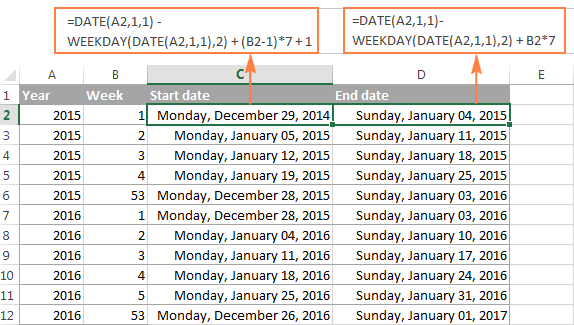#### Formula 2. A week containing Jan-1 is week 1, Sun-Sat week

These formulas are similar to the above ones with the only difference that they are written for Sunday - Saturday week.

Start date:
`=DATE(A2,1,1) - WEEKDAY(DATE(A2,1,1),1) + (B2-1)*7 + 1`

End date:
`=DATE(A2,1,1)- WEEKDAY(DATE(A2,1,1),1) + B2*7`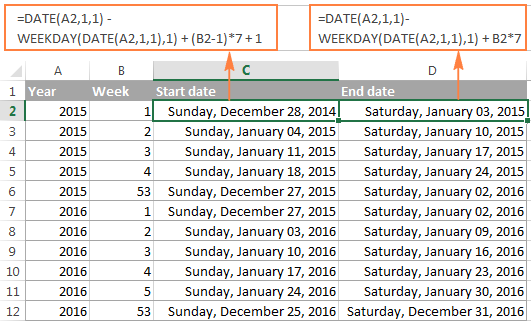#### Formula 3. Always start counting on January 1, Mon-Sun week

While the previous formulas return Monday (or Sunday) of week 1, regardless of whether if falls within this year or the previous year, this start date formula always returns January 1 as the start date of week 1 regardless of the day of the week. By analogy, the end date formula always returns December 31 as the end date of the last week in the year, regardless of the day of the week. In all other respects, these formulas work similarly to Formula 1 above.

Start date:
`=MAX(DATE(A2,1,1), DATE(A2,1,1) - WEEKDAY(DATE(A2,1,1),2) + (B2-1)*7 + 1)`

End date:
`=MIN(DATE(A2+1,1,0), DATE(A2,1,1) - WEEKDAY(DATE(A2,1,1),2) + B2*7)`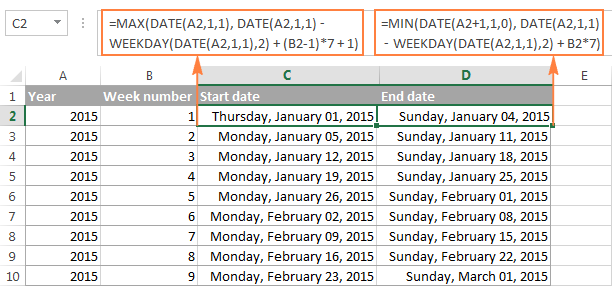#### Formula 4. Always start counting on January 1, Sun-Sat week

To calculate the start and end dates for a Sunday - Saturday week, all it takes is one small adjustment in the above formulas :)

Start date:
`=MAX(DATE(A2,1,1), DATE(A2,1,1) - WEEKDAY(DATE(A2,1,1),1) + (B2-1)*7 + 1)`

End date:
`=MIN(DATE(A2+1,1,0), DATE(A2,1,1) - WEEKDAY(DATE(A2,1,1),1) + B2*7)`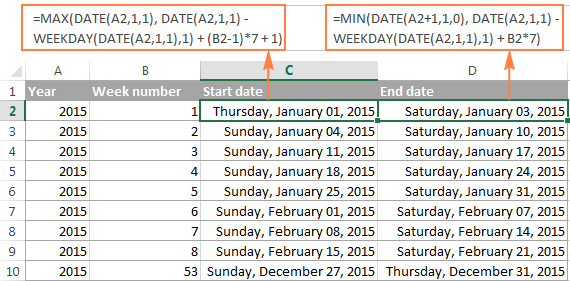## How to get month from week number

To get a month corresponding to the week number, you find the first day in a given week as explained in this example, and then wrap that formula in the Excel MONTH function like this:

`=MONTH(DATE(A2, 1, -2) - WEEKDAY(DATE(A2, 1, 3)) + B2 * 7)`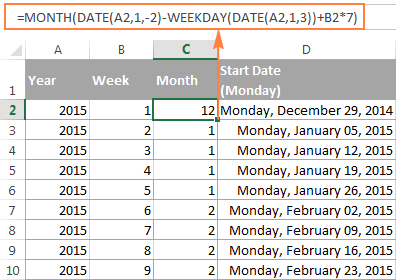Note. Please remember that the above formula works based on the ISO week date system, where the week starts on Monday and the week containing the 1st Thursday of the year is considered week 1. For example, in the year 2016, the first Thursday is January 7, and that is why week 1 begins on 4-Jan-2016.

## How to get a week number in a month (from 1 to 6)

If your business logic requires converting a specific date to the week number within the corresponding month, you can use the combination of WEEKNUM, DATE and MONTH functions:

Assuming that cell A2 contains the original date, use the following formula for a week beginning on Monday (notice 21 in WEEKNUM's return_type argument):

`=WEEKNUM(\$A2,21)-WEEKNUM(DATE(YEAR(\$A2), MONTH(\$A2),1),21)+1`

For a week beginning on Sunday, omit the return_type argument:

`=WEEKNUM(\$A2)-WEEKNUM(DATE(YEAR(\$A2), MONTH(\$A2),1))+1`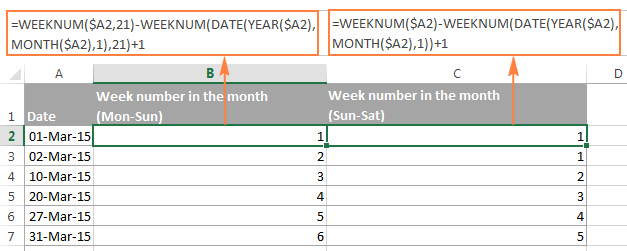## How to sum values and find average by the week number

Now that you know how to convert a date to a week number in Excel, let's see how you can use week numbers in other calculations.

Suppose, you have some monthly sales figures and you want to know the total for each week.

To begin with, let's find out a week number corresponding to each sale. If your dates are in column A and sales in column B, copy the `=WEEKNUM(A2)` formula across column C beginning in cell C2.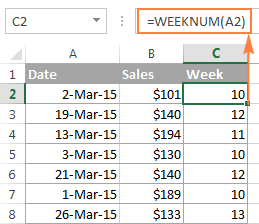And then, make a list of week numbers in some other column (say, in column E) and calculate the sales for each week using the following SUMIF formula:

`=SUMIF(\$C\$2:\$C\$15, \$E2, \$B\$2:\$B\$15)`

Where E2 is the week number.

In this example, we are working with a list of March sales, so we have week numbers 10 to 14, as demonstrated in the following screenshot: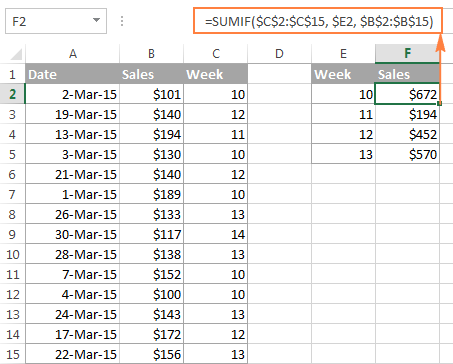In a similar manner, you can calculate the sales average for a given week:

`=AVERAGEIF(\$C\$2:\$C\$15, \$E2, \$B\$2:\$B\$15)`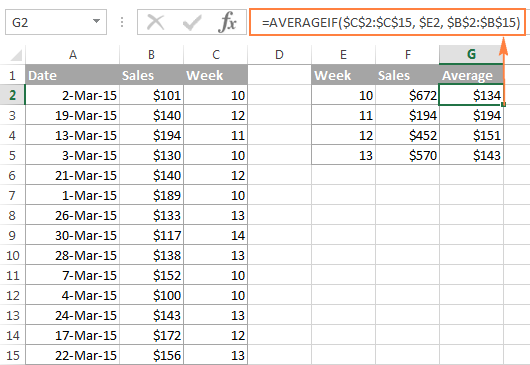If the helper column with the WEEKNUM formula does not fit well into your data layout, I regret to tell you that there is no simple way to get rid of it because Excel WEEKNUM is one of those functions that doesn't accept range arguments. Therefore, it cannot be used within SUMPRODUCT or any other array formula like the MONTH function in a similar scenario.

## How to highlight cells based on the week number

Let's say you have a long list of dates in some column and you want to highlight only those that relate to a given week. All you need is a conditional formatting rule with a WEEKNUM formula similar to this:

`=WEEKNUM(\$A2)=10`

As demonstrated in the screenshot below, the rule highlights sales that were made within week 10, which is the first week in March 2015. Since the rule applies to A2:B15, it highlights values in both columns. You can learn more about creating conditional formatting rules in this tutorial: Excel conditional formatting based on another cell value.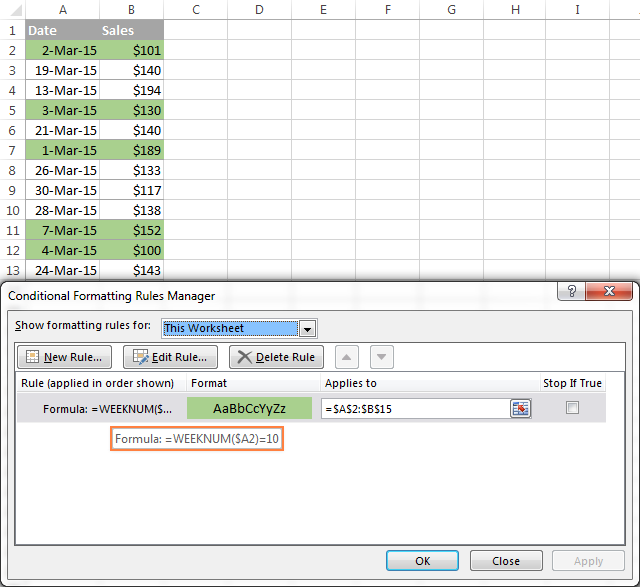This is how you can calculate week numbers in Excel, convert week number to date and extract week number from date. Hopefully, the WEEKNUM formulas you have learned today will prove useful in your worksheets. In the next tutorial, we will talk about calculating age and years in Excel. I thank you for reading and hope to see you next week!

## You may also be interested in

1. Good Afternoon ALCON,
I have three date related formulas where, if there is no data in the corresponding field, there is still a return value. Case in point: =WEEKNUM([@[Check-Out Date]],1)-40+IF(MONTH([@[Check-Out Date]])<10,53,1) will return with "13" if the "Check-Out Date" field is blank. Is there a way to adjust the formula to have the field remain blank? The other formulas in question are: =MONTH([@[Check-Out Date]])-10+IF(MONTH([@[Check-Out Date]])<10,13,1) which returns "4" and = YEAR([@[Check-Out Date]]) which returns "1900"

• Hi! I can't check and correct a formula that contains unique references to your data. To perform calculations only when a date is written in the cell, add a condition using the IF function.
For example,

IF(A1<>"", [formula],"")

2. I have Date and month in excel data. I want to get week number which 1st monday included in that month. How to get that?

• Hi! To find the date of the first Monday of the month, use the formula:

=DATE(YEAR(D1),MONTH(D1),(D3*7)+1) - WEEKDAY(DATE(YEAR(D1),MONTH(D1),8-D2),2)

D1 - any date of the month
D2 - number for the week (starting with Monday)
D3 - Nth occurrence in the month

To find the week number, add the WEEKNUM function.

=WEEKNUM(DATE(YEAR(D1),MONTH(D1),(D3*7)+1) - WEEKDAY(DATE(YEAR(D1),MONTH(D1),8-D2),2))

3. sir .
excel 2007 does not many return_type please explain how i should start Friday to Thursday ?

4. Hello all experts,

I have a task which needs to return every first and third Wednesday of each month and every second and fourth Monday of each month.

The tricky part is, we don't use the ISO week system. We simply use the first week of that month. Meaning that for example Feb 2023, 1/2/2023 is Wednesday, and this is the first week. So no first Monday will be return on that month and the second Monday should return 6 Feb 2023 and fourth Monday will be 20 Feb 2023 and so on using the same algorithm for every month and every year.

Is there any way by using formula to achieve this, please advise.

Thank you so much in advance.

5. Dear All,

I have a question. I have to make an excel function. A have a column with Year (in format 2021.01.01), a Week Nr. (in format 1-52). I have to make a third column for the following method:

Concatenating the year (from a column containg the year in format 2012.01.01),
a letter "W" and the Week Nr (a Week Nr smaller than 10 must be preceded by a "0", e.g. 2012W01).

--A made the calculation for the Week Nr (with the WEEKNUM function, the second parameter of which is: 21 (this is the ISO 8601 week number, the
week containing the first Thursday of the year is week 1)).
-- It is interesting, that the Week nr of 2012.12.31 was/is 1 week. Is it an error, or I have to make a calculation, to not repeat it (because theree is 1st
week in 2012.01.01-2012.01.8?

• Hi!
Try using parameter 2 in the WEEKNUM function.

6. How can I create a work schedule with following : 4days off , 5days on , 4 days off , 5 days on, 5days off , 4 days on , 5 days off,

For a year , the working is like that .
Ex : starting on 1 January 2023
Thank you

• Hi!
I don't see any pattern in your data. If there is, please describe.

7. Hi,
How can I convert my work week number to date or vice versa.
Example:
1) Input = 25.3, Output = 15/06/2022
2) Input = 15/06/2022 to WW25.3
here the week number starting from Monday.

• Hello!
To convert a week number to a date, try this formula with WEEKDAY function:

=MAX(DATE(YEAR(TODAY()),1,1),DATE(YEAR(TODAY()),1,1) - WEEKDAY(DATE(YEAR(TODAY()),1,1),2) + (A2-1)*7+1)

• Thank you so much, That's perfectly working.

Can you also tell us how to do in reverse.
I mean if I enter date it should give me work week number.
Example:
Input = 15/06/2022 then it should give the output as WW25.3

• Hi!
To get the number after the decimal point, use the WEEKDAY function.

=WEEKNUM(D1) + WEEKDAY(D1,2)/10

8. Please how can I calculate week 38 to months

• Hello!
To determine the month number, use the MONTH function

=MONTH(A1*7)

or

=MONTH(A1*7+DATE(YEAR(TODAY()),1,1))

Here the month number is calculated from the last day of the week.

9. Hi,
Is there a way to convert a week number (i.e 19) to a date format (1/23/2022) given current date?

Thank you so much!

• Thank you, I appreciate your help!

10. Hi!
Any ideas whether to use an ISOWEEKNUM function or a Format Cells option:
e.g. a project starts in week X, no binding to date or year.
Different activities are to be accomplished prior to the project start - let's say task A: week "X- 7" before project start, task B: week "X - 4" before, task C: week "X - 2" before, etc..
How can a project start week be determined as a week nr for excel, otherwise week 3 starting project shows "-4" for task A instead of 49.
Thanks,
Daniel

• Hi!
The week number can only be determined by the date. You have no date.

11. Hi,
My financial year starts from 1st April and whatever day it is it should be week 1 and should change to next week after Sunday. Means week is calculated from Monday to Sunday.
1st April'20 to 5 April - week1
6th April to 12 April - week 2
And further proceed in similar way till 31st March'21.
Pls suggest the formula.
TIA

• Hello!

=IF(MONTH(A2)>3,WEEKNUM(A2,2)-13,WEEKNUM(DATE(YEAR(A2),12,31),2)+WEEKNUM(A2)-13)

Please try to decrease the length of formula so beginners can understand easily. The below is example for Jul-Jun financial year.

=IF(MONTH(A2)>6,WEEKNUM(A2,2)-27,53+WEEKNUM(A2)-27)

12. Hello!
I have a scenario where we want to take the date and convert that date to say 'week of Sep 20th" which would be the Monday of that week

my date is 23-SEP-2021 - i can use "=A2-WEEKDAY(A2,2)+1" to get the Monday date
result: 20-SEP-2021
But can it be converted all at once to return "Week of Sep 20th" instead using the original date of Sep 23?

• Hello!
If I understand your task correctly, the following formula should work for you:

=CONCATENATE("Week of ",TEXT(A2-WEEKDAY(A2,2)+1,"mmm")," ", DAY(A2-WEEKDAY(A2,2)+1) & LOOKUP(DAY(A2-WEEKDAY(A2,2)+1), {1,"st";2,"nd";3,"rd";4,"th";21,"st";22,"nd";23,"rd";24,"th";31,"st"}))

13. Hello,
Could you please solve my issue. I have cell criteria, A1 = year, B1 = month and C1 = week number. My week starts on Sunday. 1 to 4 March 2021 should be the first week and 6 to 11 March 2021 should be the second week. If I change the cell value C1 = 6 than formula return should be blank. Hence, it should not bring any date from any other month.
DATE(A1,MONTH(B1),1) - WEEKDAY(DATE(A1,MONTH(B1),1),1)+ (C1-1)*7

• Sorry ,Just small correction, My week starts on Saturday

• Hello!
Specify exactly what you want to get with your formula.

14. Thank you very much for this post, very usefulc clear and detailed.

15. Thank you. I was looking for this.
I use Excel 2010 so I had to convert all the ; and also it's a Danish version So I had to change the Max to Maks, Date to Dato, and weekday to Ugedag. Then it worked brilliantly ☺

• I made a typo ☺.
I had to change all the , commas to ; semicolon for it work in Excel 2010

16. Is there a way to use =WEEKNUM(TODAY()), but have it display in the cell with additional text before and after, i want the text to stay the same, but i want the Week Number to auto update each time someone opens the spreadsheet

Week 47 Thu

• Hello!

17. I was wondering. I am not very computer / excel savvy.
I need to work out the week number from a specific date.
eg If someone has surgery on 19/8/2020 and it always based on 'todays date' what is the week number.
e.g date of surgery: 19/8/2020. today's date: 14/10/2020 ... this is 8 weeks post surgery... I need to do this in my excel spreadsheet.... thanks in advance

18. Rather is "WC 12 Oct'20" in this format

19. Hi can someone help with to set week commencing in below format

like if the week is start 12 Oct 2020 then I would like to see it as WC 12 Oct 2020

• Hello!
Sorry, I do not fully understand the task.
What does WC mean?

20. Hi,

I sincerely appreciate any help that can be offered to help me solve this problem:

I'm dealing with reports that provide only week numbers and years - I believe the week numbering system is the same as Excel's WEEKNUM type 21 (ISO, Mon-Sun). I used the formulas in the article ...

Start Date
=DATE([Year], 1, -2) - WEEKDAY(DATE([Year], 1, 3)) + ([Week Num] * 7)

End Date
=DATE([Year], 1, -2) - WEEKDAY(DATE([Year], 1, 3)) + ([Week Num] * 7) + 6

... to try to calculate the start and end date for each week, but I'm seeing obviously wrong dates being calculated at the end/beginning of each year -- the week start/end dates are wrong for 1/1/2021 through 1/3/2021.

Date Year Wk Nm Wk Start Date Wk End Date
12/21/2020 2020 52 12/21/2020 12/27/2020
12/22/2020 2020 52 12/21/2020 12/27/2020
12/23/2020 2020 52 12/21/2020 12/27/2020
12/24/2020 2020 52 12/21/2020 12/27/2020
12/25/2020 2020 52 12/21/2020 12/27/2020
12/26/2020 2020 52 12/21/2020 12/27/2020
12/27/2020 2020 52 12/21/2020 12/27/2020
12/28/2020 2020 53 12/28/2020 1/3/2021
12/29/2020 2020 53 12/28/2020 1/3/2021
12/30/2020 2020 53 12/28/2020 1/3/2021
12/31/2020 2020 53 12/28/2020 1/3/2021
1/1/2021 2021 53 1/3/2022 1/9/2022
1/2/2021 2021 53 1/3/2022 1/9/2022
1/3/2021 2021 53 1/3/2022 1/9/2022
1/4/2021 2021 1 1/4/2021 1/10/2021
1/5/2021 2021 1 1/4/2021 1/10/2021
1/6/2021 2021 1 1/4/2021 1/10/2021
1/7/2021 2021 1 1/4/2021 1/10/2021
1/8/2021 2021 1 1/4/2021 1/10/2021
1/9/2021 2021 1 1/4/2021 1/10/2021
1/10/2021 2021 1 1/4/2021 1/10/2021

Is there an improved version of the formula that will correctly calculate the week start/end dates for between year transitions?

Thank you.

Respectfully,
Mark

• I just realized my mistake -- I'm deriving the year from the date, rather than from the weekly period, which would be 2020-53, not 2021-53, for 1/1/2021 through 1/3/2021.

• Hello!
The WEEKNUM function can use 2 counting systems for the first week of the year. See the syntax for this function in more detail and choose the system that works best for you.
I hope this will help, otherwise please do not hesitate to contact me anytime.

21. This is an amazing post. Saved many hours of my life! Sincerely appreciate the hard work you have put in and your willingness to share.
Best regards,
Junaid.

22. Can anyone explains to me the logic behind the formula:
date - WEEKDAY(date - 2)?
It is doing my head in.